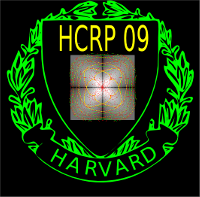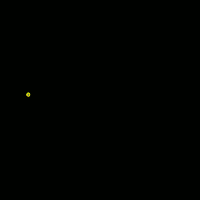HCRP project 2009
HCRP spring 2009 Project
Caustics in Differential Geometry
Office: SciCtr 434
 This is a continuation of a fall 2008 project, made possible by the HCRP (Harvard College Research Program). Some interesting questions have emerged in the field of differential geometry, which are exciting not only from the mathematical point of view, but also because they reach other fields.The problem is at the heart of differential geometry: given a point on a space, one can send out light from that point. Light rays move on geodesic paths, curves which locally minimize arc length. While the wave front - the set of points which are reached after some time - looks first like a sphere, it will be distorted over time, and eventually change topology. Places, where fold-overs happen, define a set called the caustic. On points of the caustic, light gets focused. One can see such caustics at the bottom of a swimming pool or in pictures from galaxies taken from a telescope. In the swimming pool case, the water surface has bent the light and the intersection of the caustic with the pool floor is seen, in the astronomy case, it is gravitational lensing which produces caustics to intersect the photo plate. In the case of seismic waves emerging from an epicenter, caustics are points, where the destruction of the waves are maximal, that is where most of the damage occurs. The caustic set is determined the metric on the space under consideration as well as an initial point. Even in very simple situations, the geometry of caustics is widely unexplored and expected to be complicated.Especially numerical computations which determine the caustic beyond the so called "cut loci", seem rare. On a compact surface, the caustic is likely to be dense on the surface if the geodesic flow is not integrable. But even on one of the simplest possible situations, the ellipsoid, the nature of the caustics is not settled. The unproven last geometric statement of Jacobi claims that except for umbillical points, the caustics has 4 cusps. A small poster of Michael about this. In our previous HCRP project we started to look at the geometry of caustics in the case of compact perturbations of the flat metric and uniform hyperbolic metric. Mathematically, we have explored the exponential map in nontrivial but accessible situations. In both these cases, the geometry of caustics can be explored with multivariable calculus methods alone because the geodesic paths are lines or circles eventually. Here, we describe caustics using Sturm-Liouville type differential equations f'' + V(t) f = 0, called Gauss-Jacobi field equations. The potential V(t) is the curvature at the light ray position at time t. This differential equation allows a convenient numerical exploration of the caustics by looking at the roots of the Jacobi field along each geodesic. Differential equations of this type occur in other areas of mathematical physics, like quantum mechanics and solid state physics. We not only want to explore the math and see experiments, but try to present the material to a larger audience. See the exhibits page.